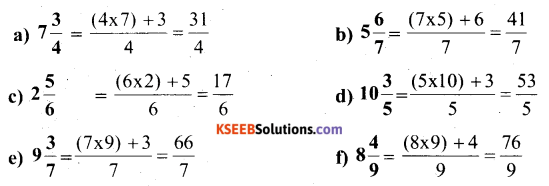# KSEEB Solutions for Class 6 Maths Chapter 7 Fractions Ex 7.2

Students can download Chapter 7 Fractions Ex 7.2 KSEEB Solutions for Class 6 Maths helps you to revise the complete syllabus.

## Karnataka State Syllabus Class 6 Maths Chapter 7 Fractions Ex 7.2

Question 1.
Draw number lines and locate the points on them: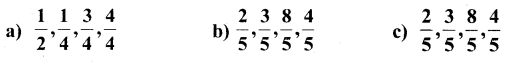Solution: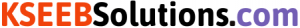Question 2.
Express the following as mixed fraction: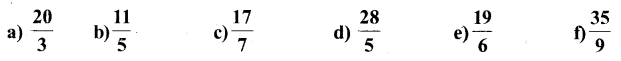Solution: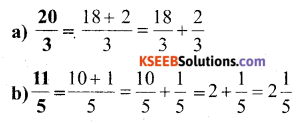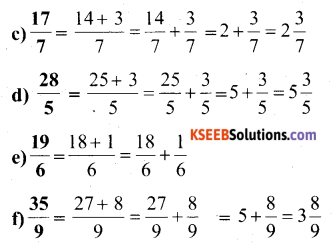Question 3.
Express the following as improper fractions: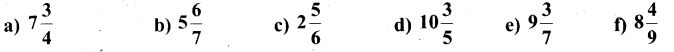Solution: